# Construct a binary search tree from a sorted linked list

In this article, we are going to see how to create a binary search tree from a sorted single linked list?
Submitted by Radib Kar, on September 22, 2020

In this article, we are going to see how we can create a height-balanced binary Search tree from a given sorted linked list.  Pay attention to the word "height-balanced" as it plays a huge role. We can create a binary search tree with the list by just creating a skew tree, where we will just put the list nodes as a right child only. For example,

Let's say the sorted list is: 1->2->3->4->5

Then the skew tree that can be created from this would be: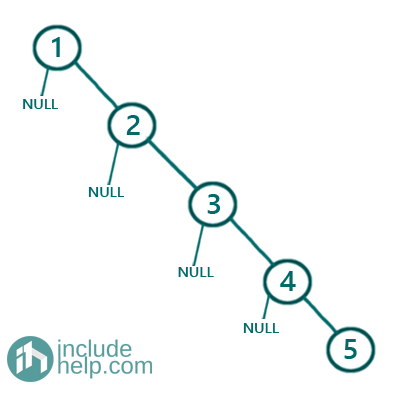Though this is a Binary Search tree, it's not what we are expecting as it's not height-balanced. For a height-balanced tree, the difference between the left & right subtree will be maximum 1. So below is the approach to create a height-balanced binary search tree from a sorted linked list.

1. Find the middle of the sorted list( Can be done with Floyd's hair & tortoise algorithm which uses a slow pointer & a fast pointer)
2. The middle node is the root, left part of the middle node is the left subtree & right part is the right subtree
3. Recursively construct left subtree & right subtree.

## To find the middle of the linked list

We use Floyd's tortoise & hare algorithm for partitioning the linked list into two halves. We declare a slow pointer and a fast pointer. The slow pointer moves one step ahead of where the fast pointer moves two-step at a time. Thus when the fast pointer reaches the end of the list, the slow pointer is at the midway.

```slow=head;

while(fast->next){
//move one step
slow=slow->next;
//move two steps if possible
fast=fast->next;
if(fast->next)
fast=fast->next;
}
//reach previous node to thee slow pointer to
//delink the left part of the slow pointer
while(temp->next!=slow){
temp=temp->next;
}
temp->next=NULL;
```

To illustrate the algorithm visually, below is the step by step pictorial representation:

Firstly the list is 1->2->3->4->5->NULL

So we find the middle and de-link the left & right part and create a root node from the middle node of the sorted linked list.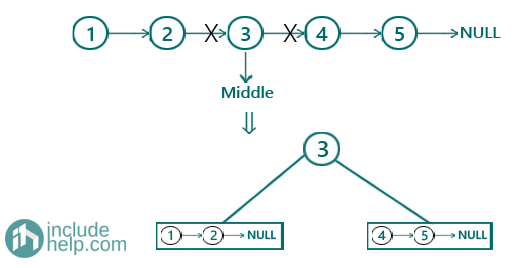Now we recursively create the left subtree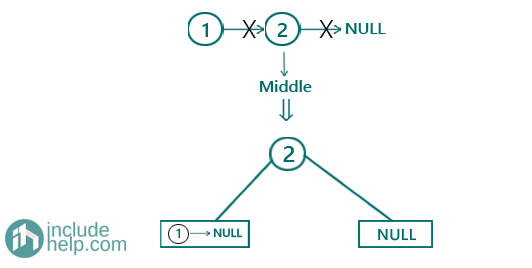Now 1->NULL a single node and that would return a leaf node in the tree whereas NULL will be NULL in the tree as well. So after constructing the left subtree the semi-constructed BST would be: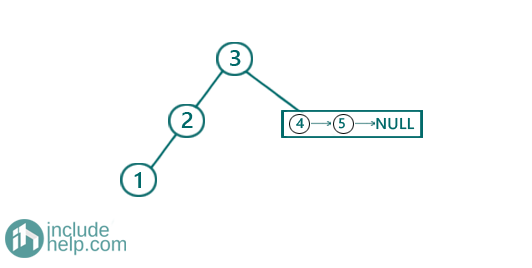Now, creating the right subtree similarly,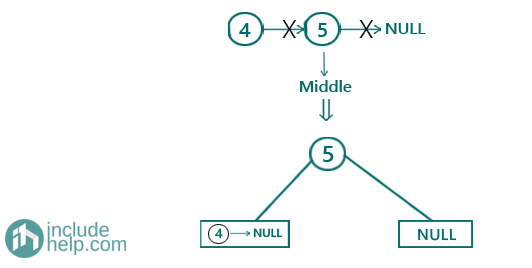Then finally the BST would be: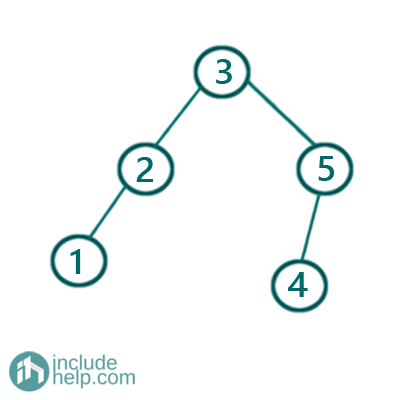N.B: this a height-balanced BST, but not only one. You can create another BST out of this which is still height-balanced and that would be like below: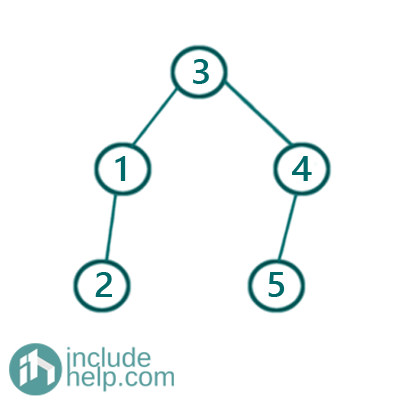Actually, it's due to the middle element we are picking up. In case of an odd length list, there is no problem, but in case of an even list there is two middle elements and based on which one we pick, there can be many such combinations. In our implementation, we have always chosen the second middle element in case of an even list as our middle element to create the root.

Below is the C++ implementation:

```#include <bits/stdc++.h>
using namespace std;

// tree node is defined
class TreeNode {
public:
int val;
TreeNode* left;
TreeNode* right;
TreeNode(int data)
{
val = data;
left = NULL;
right = NULL;
}
};

class ListNode {
public:
int val;
ListNode* next;
ListNode(int data)
{
val = data;
next = NULL;
}
};

void inorder(TreeNode* root)
{

if (!root)
return;
inorder(root->left);
cout << root->val << " ";
inorder(root->right);
}

{
//base cases
//1.NULL list
return NULL;
//2. single node list

//find the middle of the linked list
//using floyd's hair & tortoise algorithm

while (fast->next) {
//slow pointer moves one step at each iteration
slow = slow->next;
//fast pointer moves two steps at each iteration
fast = fast->next;
//this checking ensures that the 2nd move is possible
if (fast->next)
fast = fast->next;
}

//reach the previous node of the current slow pointer
while (temp->next != slow) {
temp = temp->next;
}
//delink the left part of slow pointer(middle of the list)
temp->next = NULL;
//create root with the middle node
TreeNode* root = new TreeNode(slow->val);
//right part of the middle node
ListNode* ptr = slow->next;
slow->next = NULL;
//recursively create left subtree from the left part
//recursively create left subtree from the right part
root->right = sortedListToBST(ptr);

return root;
}

int main()
{
//building the tree like the example

cout << "Creating self-balancing BST from the sorted list\n";

cout << "height balanced BST created ...\n";
cout << "root of the BST created is: " << root->val << endl;
cout << "Inorder traversal of the BST\n";
inorder(root);
cout << endl;

return 0;
}
```

Output:

```Creating self-balancing BST from the sorted list
height balanced BST created ...
root of the BST created is: 3
Inorder traversal of the BST
1 2 3 4 5
```

Dry run two show how Floyd's hair & tortoise works

Iteration 0 (Initially):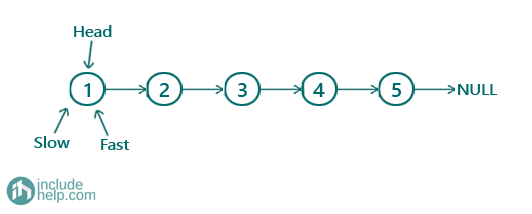Iteration 1: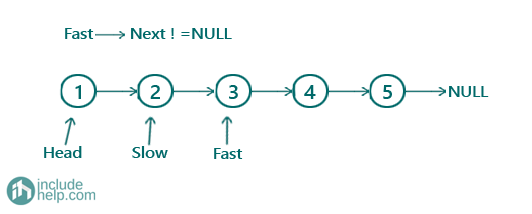Iteration 2: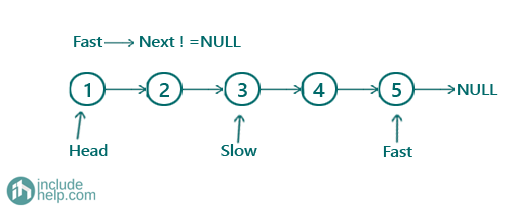Iteration 3:So now wherever slow pointer points that' our middle node which is 3 here

Now to delink the left part & right part we traverse a temp to the previous node of slow and then delink the left part & delinking right part is as simple as slow->next=NULL after we save the right part to some other pointer (otherwise we will lose the right part).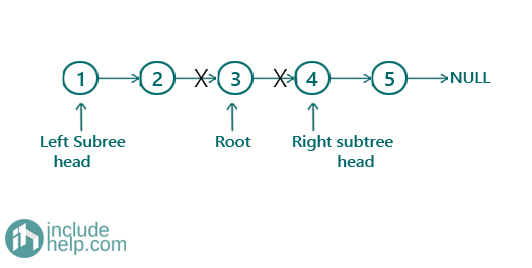Top MCQs